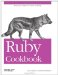# Rearranging Values Without Using Temporary Variables

Problem

You want to rearrange a number of variables, or assign the elements of an array to individual variables.

Solution

Use a single assignment statement. Put the destination variables on the left-hand side, and line each one up with a variable (or expression) on the right side.

A simple swap:

```	a = 1
b = 2
a, b = b, a
a # => 2
b # => 1
```

A more complex rearrangement:

```	a, b, c = :red, :green, :blue
c, a, b = a, b, c
a # => :green
b # => :blue
c # => :red
```

You can split out an array into its components:

```	array = [:red, :green, :blue]
c, a, b = array
a # => :green
b # => :blue
c # => :red
```

You can even use the splat operator to extract items from the front of the array:

```	a, b, *c = [12, 14, 178, 89, 90]
a # => 12
b # => 14
c # => [178, 89, 90]
```

Discussion

Ruby assignment statements are very versatile. When you put a comma-separated list of variables on the left-hand side of an assignment statement, it's equivalent to assigning each variable in the list the corresponding right-hand value. Not only does this make your code more compact and readable, it frees you from having to keep track of temporary variables when you swap variables.

Ruby works behind the scenes to allocate temporary storage space for variables that would otherwise be overwritten, so you don't have to do it yourself. You don't have to write this kind of code in Ruby:

```	a, b = 1, 2
x = a
a = b
b = x
```

The right-hand side of the assignment statement can get almost arbitrarily complicated:

```	a, b = 5, 10
a, b = b/a, a-1 # => [2, 4]

a, b, c = 'A', 'B', 'C'
a, b, c = [a, b], { b => c }, a
a # => ["A", "B"]
b # => {"B"=>"C"}
c # => "A"
```

If there are more variables on the left side of the equal sign than on the right side, the extra variables on the left side get assigned nil. This is usually an unwanted side effect.

```	a, b = 1, 2
a, b = b
a # => 2
b # => nil
```

One final nugget of code that is interesting enough to mention even though it has no legitimate use in Ruby: it doesn't save enough memory to be useful, and it's slower than doing a swap with an assignment. It's possible to swap two integer variables using bitwise XOR, without using any additional storage space at all (not even implicitly):

```	a, b = rand(1000), rand(1000) # => [595, 742]
a = a ^ b # => 181
b = b ^ a # => 595
a = a ^ b # => 742

[a, b] # => [742, 595]
```

In terms of the cookbook metaphor, this final snippet is a dessertno nutritional value, but it sure is tasty.Ruby Cookbook (Cookbooks (OReilly))
ISBN: 0596523696
EAN: 2147483647
Year: N/A
Pages: 399